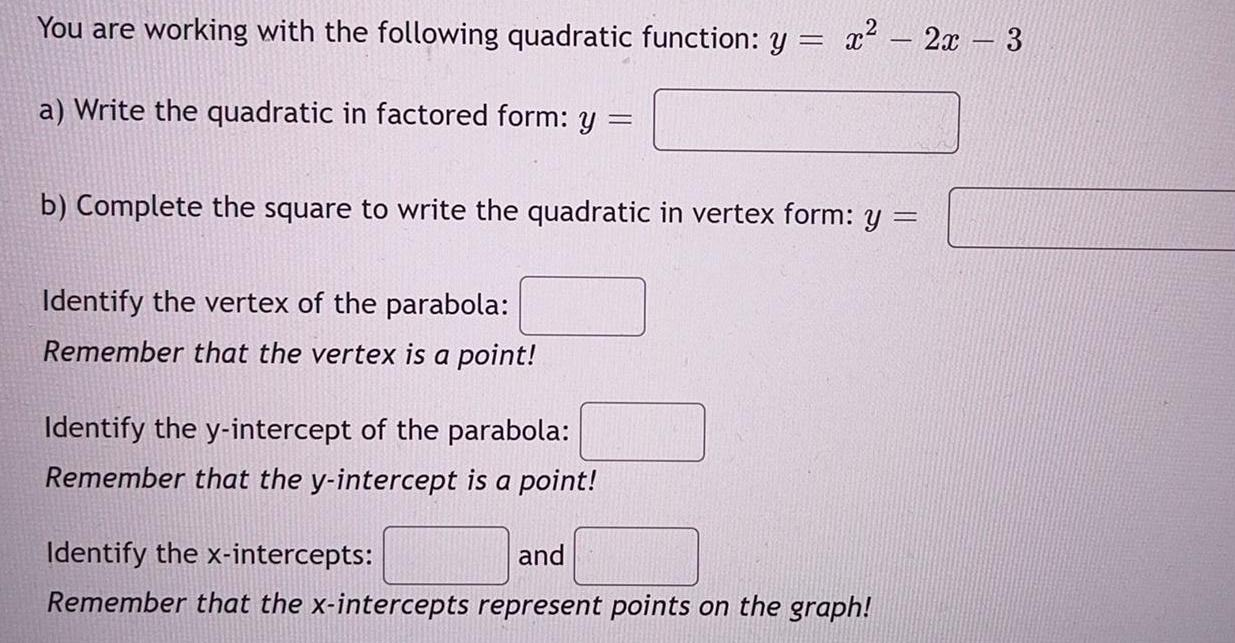Question:

# You are working with the following quadratic function

Last updated: 8/26/2022You are working with the following quadratic function: y = x² - 2x - 8 a) Write the quadratic in factored form: y= b) Complete the square to write the quadratic in vertex form: y = Identify the vertex of the parabola: Remember that the vertex is a point! Identify the y-intercept of the parabola: Remember that the y-intercept is a point! Identify the x-intercepts: and Remember that the x-intercepts represent points on the graph!